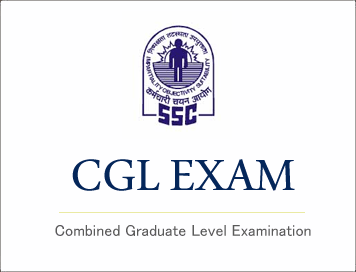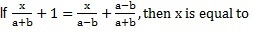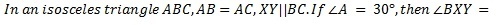# SSC CGL (Tier - 1) Online Exam Paper - 2016 "held on 7 September 2016" Morning Shift (Quantitative Aptitude)## SSC CGL (Tier - 1) Online Exam Paper - 2016 "held on 7 September 2016" Morning Shift (Quantitative Aptitude)

EXAM DATE : 7-September-2016
EXAM START TIME :
10:00:00
EXAM NAME :
SSC Examination 2016

Question 51.Find the least number which must be subtracted from 18265 to make it a perfect square

Options:

1) 30
2
) 38
3
) 40
4
) 45

Question 52.A and B together can do a piece of work in 36 days, B and C together can do it in 24 days. A and C together can do it in 18 days. The three working together can finish the work in

Options:

1) 8 days
2) 16 days
3) 30 days
4) 32 days

Correct Answer: 16 days

Question 53.Two adjacent sides of a parallelogram are 21 cms and 20 cms. The diagonal joining the end points of these two sides is 29 cms. The area of the parallelogram (in sq.cms) is

Options:

1) 240
2
) 120
3
) 210
4
) 420

Question 54.A dealer marks his goods 20% above cost price. He then allows some discount on it and makes a profit of 8%. The rate of discount is

Options:

1) 4%
2) 6%
3) 10%
4) 12%

Question 55.Sum of two numbers is thrice their difference. Their ratio is

Options:

1) 1:2
2
) 2:1
3
) 3:1
4
) 1:3

Question 56.The average age of 36 students in a group is 14 years. When the teacher's age is included in it, the average increases by one. The teacher's age in years is

Options:

1) 31
2
) 51
3
) 36
4
) 50

Question 57.A dishonest dealer professes to sell his goods at cost price but uses a weight of 875 gms for the kilogram weight. His gain in percentage isQuestion 58.A's salary is 50% more than that of B. Then B's salary is less than that of A by## Study Kit for SSC CGL EXAM

Question 59.Speed of a boat along and against the current are 14 kms/hr and 8 kms/hr respectively. The speed of the current is

Options:

1) 11 kms/hr
2) 6 kms/hr
3
) 5.5 kms/hr
4) 3 kms/hr

Correct Answer: 3 kms/hr

Question 60.If the simple interest on Rs. 1 for 1 month is 1 paisa, then the rate percent per annum will be

Options:

1) 10%
2
) 8%
3
) 12%
4
) 6%

Question 61.If a/b + b/a = 1, then the value of a3 + b3will be

Options:

1) 1
2
) 0
3
) -1
4) 2

Question 62.If p = 99, then the value of p(p2+3p+3) will be

Options:

1) 999999
2
) 1000000
3
) 1000001
4
) 999998

Question 63.If a-b=1 and a3-b= 61, then the value of ab will be

Options:

1) -20
2
) 20
3
) 30
4
) 60

Question 64.Options:

1) 2a-b
2
) a+b
3) a-b
4
) 2a+b

Question 65.The point where the 3 medians of a triangle meet is called

Options:

1) centroid
2) Incentre
3) Circumcentre
4) orthocentre

Question 66.Δ ABC a right angled triangle has ∠B = 90° and AC is hypotenuse. D is its circumcentre and AB = 3 cms, BC = 4 cms. The value of BD is

Options:

1) 3cms
2) 4 cms
3) 2.5 cms
4) 5.5 cms

Correct Answer: 2.5 cms

Question 67.Δ ABC is an equilateral triangle and D, E are midpoints of AB and BC respectively. Then the area of Δ ABC : the area of the trapezium ADEC is

Options:

1) 5:3
2
) 4:1
3
) 8:5
4
) 4:3

Question 68.Options:

1) 75°
2
) 30°
3
) 150°
4
) 105°

Question 69.Options:

1) 1
2
) 0
3
) -1
4
) 2

Question 70.If x=aCosθCosΦ, y = aCosθSinΦ and z= aSinθ, then the value of x2+ y2 + z2 is

Options:

1) 2a2
2) 4a2
3) 9a2
4) a2

Question 71.A 1.6 m tall observer is 45 meters away from a tower. The angle of elevation from his eye to the top of the tower is 30°, then the height of the tower in meters is
(Take √3 = 1.732)

Options:

1) 25.98
2
) 26.58
3) 27.58
4) 27.98

Question 72.The following pie chart shows proportion of population of seven villages in 2009. Study the pie chart and answer the questions that follows :If the below poverty line population of the village 'X' is 12160, then the population of village 'S' is

Options:

1) 18500
2) 20500
3) 22000
4) 20000

Question 73.The following pie chart shows proportion of population of seven villages in 2009. Study the pie chart and answer the questions that follows :The ratio of the below poverty line population of village 'T' to that of the below poverty line population of village 'Z' is

Options:

1) 11:23
2
) 13:11
3
) 23:11
4) 11:13

Question 74.The following pie chart shows proportion of population of seven villages in 2009. Study the pie chart and answer the questions that follows :If the population of the village 'R' is 32000, then the below poverty line population of village 'Y' is

Options:

1) 14100
2) 15600
3) 16500
4) 17000

Question 75.The following pie chart shows proportion of population of seven villages in 2009. Study the pie chart and answer the questions that follows :In 2010, the population of 'Y' and 'V' increases by 10% each and the percentage of population below poverty line remains unchanged for all the villages. If in 2009, the population of village Y was 30,000, then the below poverty line population of village 'V' in 2010 is _____

Options:

1) 11250
2
) 12760
3
) 13140
4
) 13780